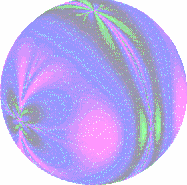We study optimisation algorithms for quadratic and convex programming. The main idea of the study is based on the renormalisation of the space. Even in simple cases, algorithms that are simply convergent can be considered as dynamic systems, after a suitable transformation is applied at each iteration. The main idea is to renormalize the region containing the search object x, for instance the point where a given function f(.) is optimum, so that the target remains in a standard region X. Therefore x is no longer fixed but moves in X at each iteration, and follows the evolution of a dynamic system. Methods of analysis relying on ergodic theory and chaos thus apply. Various algorithms are considered, e.g. line search, ellipsoid, steepest descent. The associated dynamic systems show different features: in some cases the asymptotic behaviour depends on x and/or on the function f(.), and periodic and strange attractors are typical. A main purpose is to use the theory of dynamic systems to analyse the asymptotic behaviour of the algorithms, and, when possible, to obtain optimal rates of convergence.

For the problem of quadratic programming in high dimensions, simple and robust gradient algorithms have been developed that are as efficient as the celebrated conjugate gradient algorithm which requires computations with infinite precision.

Applications to diverse problems of applied mathematics are under consideration.

Cardiff investigators

• Prof Anatoly Zhigljavsky
• Dr Rebecca Haycroft

Collaborators

• Dr Luc Pronzato
• Prof Henry Wynn

Selected publication

• Pronzato L., Wynn, H.P., Zhigljavsky, A. (2000) Dynamical search. Applications of dynamical systems in search and optimization. Chapman & Hall/CRC, Boca Raton, FL. xii+221 pp.

• Haycroft R., Pronzato L., Wynn H.P., Zhigljavsky A.A. (2008) Studying Convergence of Gradient Algorithms via Optimal Experimental Design Theory, In search for optimality in design and statistics (L. Pronzato and A. Zhigljavsky, eds.), Springer-Verlag, pp. 13-38.
• Pronzato L., Wynn H.P., Zhigljavsky A.A. (2006) Asymptotic behaviour of a family of gradient algorithms in R^ d and Hilbert spaces. Math. Program. 107, no. 3, Ser. A, 409-438.
• Pronzato L., Wynn H.P., Zhigljavsky A.A. (2005) Kantorovich-type inequalities for operators via D-optimal design theory. Linear Algebra Appl., 410, 160-169.
• Pronzato L., Wynn H.P., Zhigljavsky A.A. (1998) A generalised Golden-Section algorithm for line--search, IMA Journal on Math. Control and Information, 15, 185-214.